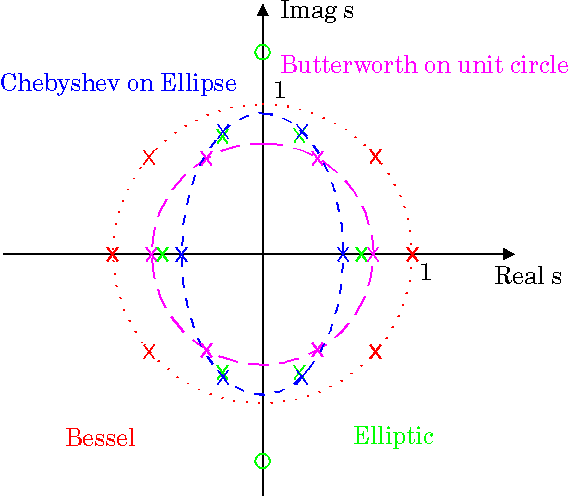# Filter shape from poles and zeros

Hey guys,

I am learning about the filter shapes deduced from the poles and zero plots, however alot of sources are describing it differently with no logical explanation. I want to know what exactly the poles and zeros do between the range Ω = 0 and Ω = π. One source i read explains if the pole is close to Ω = 0 the magnitude will be large hence a low pass behaviour...to be honest i wasn't quite sure how that came to that conclusion.

If you guys can simply explain how the filter shapes are brought about for Low pass, high pass, bandstop and bandpass it will be much appreciated.

Thanks!

I think, it is not easy to answer because we don`t know the level of your knowledge.
* For example, do you know the "secrets" behind the complex variable "s" ?
* Do you know why sometimes s=σ+jω and sometimes s=jω ?
* Do you know what happens with the magnitude of a transfer function at the pole or at the zero frequency, respectively?
* What is the meaning of Ω = π ?

Last edited:
meBigGuy
Gold Member
You can, I assume, solve for the poles and zeros. Let's call the poles and zeros "break points", in that the gain slope "breaks (changes) at those points.

Write down the frequencies of all the break points in order. Draw a plot starting at zero.

Gain increases at 20dB/decade at each zero, and decreases at 20dB/decade at each pole.

If the poles and zeros do not interact, and are not complex, then this is pretty close.

For example, a pole at F1 will have 0 gain gain change until F1, after which its gain will decrease at 20dB/decade. A zero at a higher frequency F2 will level the gain back to 0 slope. (The true gain is 3dB off the breakpoints)

The following paper explains the above, and also addresses more complex scenarios:
http://my.ece.ucsb.edu/York/Bobsclass/2B/Frequency Response.pdf

So basically on a real and imaginary axis you would plot the poles and zeros that are found from the numerator and the denominator. Poles are represented as X and zeros are represented as o. Just by looking at a graph i want to be able to observe what type of filter it is, which is what im having trouble with.

So basically on a real and imaginary axis you would plot the poles and zeros that are found from the numerator and the denominator.

No - you have to discriminate between the complex s-plane (showing the poles as "x", for,example) and the BODE diagram - consisting of a plot showing the MAGNITUDE and the PHASE as a function of frequency (see the referenced links).

Im not sure if bode diagrams are relevant, this topic i'm doing is on digital signal processing. Really i just need some conditions on the poles and zeros for different types of filters

meBigGuy
Gold Member
Basically as you traverse the unit circle from 0 to 2*pi in the Z plane ( or the jw axis in the s-plane) you can consider the distances to the poles or zeros for their effect on that frequency.

Don't get mixed up whether you are viewing s plane or z plane.

The difference between pole zero plots for digital (Z) or continuous filters (S plane) is whether you traverse the unit circle (Z plane) or the jw axis (s-plane).

The amplitude at a frequency is the product of magnitude of the vectors from that point to all zeros divided by product of magnitude of the vectors to all poles

Generally the filter is designed in the S-plane and then transformed to a digital equivalent (by bilinear transform or whatever)

There are lot of examples of pole zero positions on the s-plane in google images. Just look up the filter type, for example
chebyshev filter pole zero s plane
chebyshev filter pole zero z plane

Here is an s plane example.